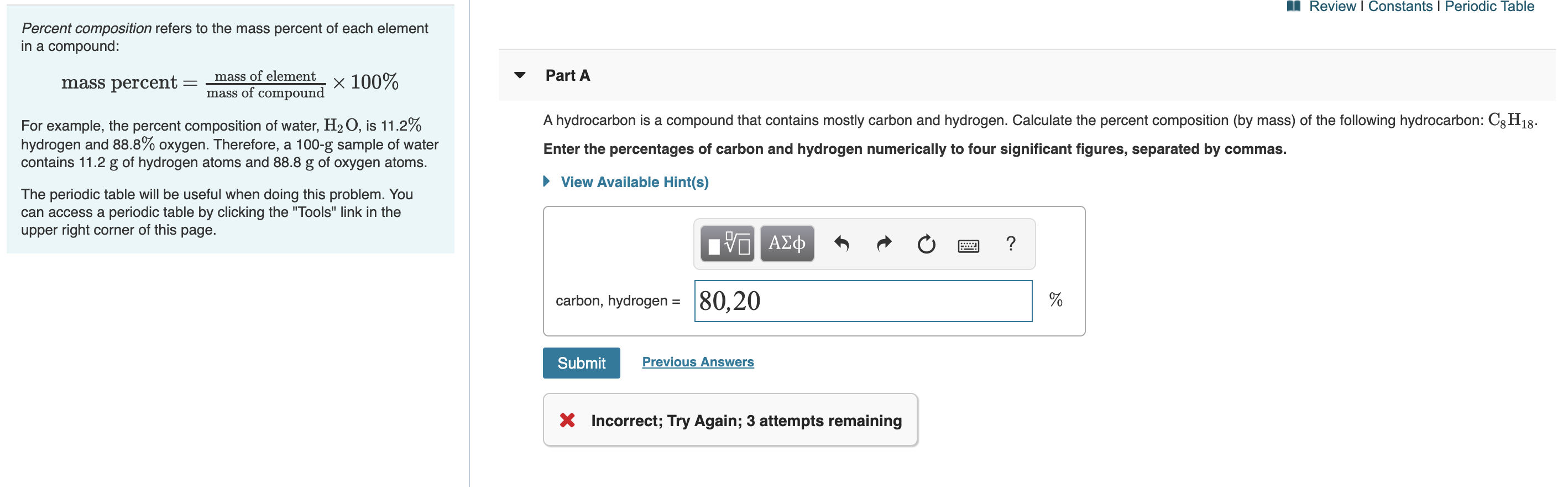# Review I Constants I Periodic Table Percent composition refers to the mass percent of each element in a compound: Part A mass of element V mass percent mass of compound X 100% A hydrocarbon is a compound that contains mostly carbon and hydrogen. Calculate the percent composition (by mass) of the following hydrocarbon: C8H18 For example, the percent composition of water, H2O, is 11.2% hydrogen and 88.8% oxygen. Therefore, a 100-g sample of water contains 11.2 g of hydrogen atoms and 88.8 g of oxygen atoms. Enter the percentages of carbon and hydrogen numerically to four significant figures, separated by commas. View Available Hint(s) The periodic table will be useful when doing this problem. You can access a periodic table by clicking the "Tools" link in the upper right corner of this page. α ΑΣφ ? |80,20 carbon, hydrogen = Previous Answers Submit X Incorrect; Try Again; 3 attempts remaining

Questionhelp_outlineImage TranscriptioncloseReview I Constants I Periodic Table Percent composition refers to the mass percent of each element in a compound: Part A mass of element V mass percent mass of compound X 100% A hydrocarbon is a compound that contains mostly carbon and hydrogen. Calculate the percent composition (by mass) of the following hydrocarbon: C8H18 For example, the percent composition of water, H2O, is 11.2% hydrogen and 88.8% oxygen. Therefore, a 100-g sample of water contains 11.2 g of hydrogen atoms and 88.8 g of oxygen atoms. Enter the percentages of carbon and hydrogen numerically to four significant figures, separated by commas. View Available Hint(s) The periodic table will be useful when doing this problem. You can access a periodic table by clicking the "Tools" link in the upper right corner of this page. α ΑΣφ ? |80,20 carbon, hydrogen = Previous Answers Submit X Incorrect; Try Again; 3 attempts remaining fullscreen

1 Rating

### Want to see this answer and more?

Experts are waiting 24/7 to provide step-by-step solutions in as fast as 30 minutes!*